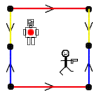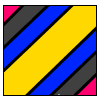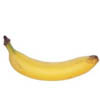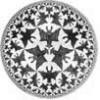# Search by Topic

Filter by: Content type:
Age range:
Challenge level:

### There are 10 results

Broad Topics > 3D Geometry, Shape and Space > Non Euclidean Geometry### Weird Universes

##### Age 16 to 18 Challenge Level:

Consider these weird universes and ways in which the stick man can shoot the robot in the back.### Torus Patterns

##### Age 16 to 18 Challenge Level:

How many different colours would be needed to colour these different patterns on a torus?### Curvature of Surfaces

##### Age 16 to 18

How do we measure curvature? Find out about curvature on soccer and rugby balls and on surfaces of negative curvature like banana skins.### Over the Pole

##### Age 16 to 18 Challenge Level:

Two places are diametrically opposite each other on the same line of latitude. Compare the distances between them travelling along the line of latitude and travelling over the nearest pole.### Spherical Triangles on Very Big Spheres

##### Age 16 to 18 Challenge Level:

Shows that Pythagoras for Spherical Triangles reduces to Pythagoras's Theorem in the plane when the triangles are small relative to the radius of the sphere.### Pythagoras on a Sphere

##### Age 16 to 18 Challenge Level:

Prove Pythagoras' Theorem for right-angled spherical triangles.### Flight Path

##### Age 16 to 18 Challenge Level:

Use simple trigonometry to calculate the distance along the flight path from London to Sydney.### When the Angles of a Triangle Don't Add up to 180 Degrees

##### Age 14 to 18

This article outlines the underlying axioms of spherical geometry giving a simple proof that the sum of the angles of a triangle on the surface of a unit sphere is equal to pi plus the area of the. . . .### How Many Geometries Are There?

##### Age 16 to 18

An account of how axioms underpin geometry and how by changing one axiom we get an entirely different geometry.### Geometry and Gravity 1

##### Age 11 to 18

This article (the first of two) contains ideas for investigations. Space-time, the curvature of space and topology are introduced with some fascinating problems to explore.